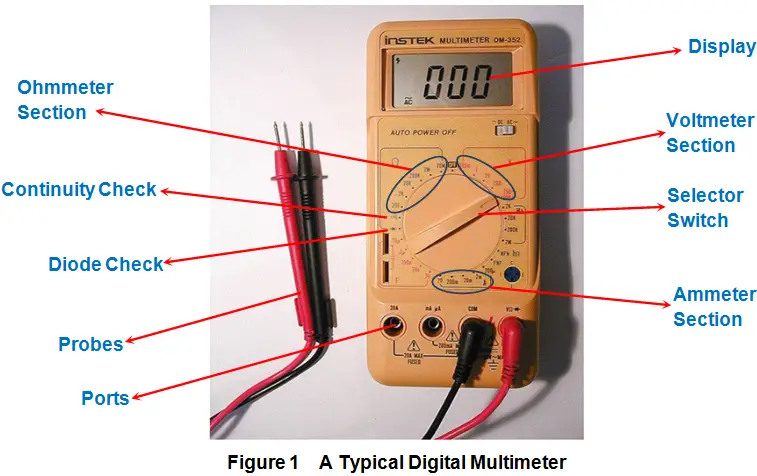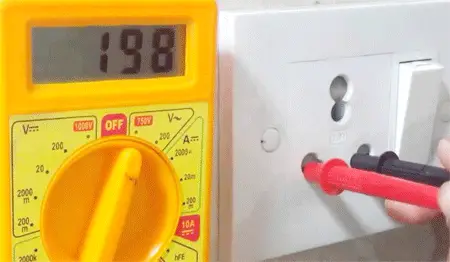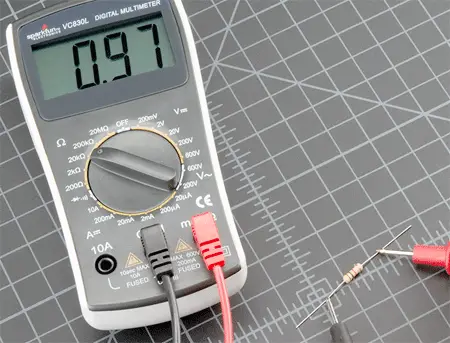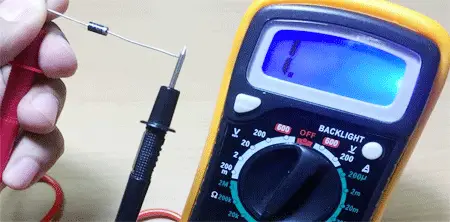# How to Use a Digital Multimeter?

Digital Multimeter is a device which has two words in its name: Digital and Multimeter. Let’s first try to analyze why do we have them there i.e. what do each of them actually convey which in turn helps us understand what does a multimeter do. The first word – digital – indicates that the meter has a digital or liquid crystal display while the next word – multimeter – indicates that this single device can be used for multipurpose i.e. to measure more than one parameter. A typical digital multimeter will be as shown by Figure 1 and comprises of selection switch, display, ports and probes as its major parts.
Here the probes are to be inserted into appropriate ports and are to be connected across the parameter which needs to be inspected. Meanwhile one should ensure that the selection switch is to be kept at the position which is appropriate for the measurement. When done so, the multimeter displays the value of the parameter which is being analyzed.Generally digital multimeters are used to measure three important parameters viz., current, voltage and resistance. Apart from these, they can also be used to perform special functions like diode check, capacitance measurement, Transistor hFE or DC current gain, frequency measurement and continuity check. In this article, we present a brief note on the most frequently used applications of multimeter which are for current, voltage and resistance measurement along with diode and continuity checks.

## Current Measurement by Using a Digital Multimeter

Under this category, the digital multimeter mimics the behavior of an ammeter as it is used to measure current. To accomplish this, insert the red probe of the multimeter to one of the current measuring sockets: mA (to measure low level of current) or 20 A (to measure larger current). Connect the meter along the line through which the current is to be measured (nothing but series connection). Next set an approximate range around which we expect the current to be in the ammeter section of Figure 1. In this state, if we switch on the power supply, then the meter will read the current flowing through the circuit.

## Voltage Measurement by Using a Digital Multimeter

When set to measure the voltage, the multimeter acts like a voltmeter. To start with, one has to insert the red and the black probes of the multimeter to the sockets marked as ‘V’ and ‘COM’, respectively. Then we have to select the expected range in which our voltage would be. Simultaneously, even AC or DC should also be selected in the voltmeter section of Figure 1. On doing so, the meter reads the value of the voltage, provided one connects the leads across the component (in parallel fashion) or at the point at which the voltage needs to be measured.## Resistance Measurement by Using a Digital Multimeter

In this case, we configure the multimeter to act like an ohmmeter. Here the red and the black probes of the multimeter are inserted into the sockets marked as ‘V’ and ‘COM’, respectively while the selection switch is set to an expected range in ohmmeter region (Figure 1). Now, the leads need to be connected across the component whose resistance is to be known. On doing so, we get a reading in the display part of the multimeter which reads the value of the resistance.## Diode Check by Using a Digital Multimeter

For this case, insert the probes into the sockets as that in the case of voltage measurement and set the selection switch to point towards diode check position shown in Figure 1. Now when the red lead of the multimeter is connected to positive terminal of the diode while its negative lead is connected to the negative terminal of the diode, then we have to get a low reading on the multimeter. On the other hand, if we connect the red lead to the negative terminal of the diode and the black to the positive terminal, then we have to get a high value. If the readings obtained are as per our expectation, then we say that the diode is working properly; else no. More information regarding this can be found in the article “Diode testing”.## Continuity Check by Using a Digital Multimeter

Continuity check is used to know whether there exists any low resistance path via two points i.e. to check whether the points are short or not. To accomplish this task, the probes are inserted into the sockets as that in the case of voltage measurement and selector switch is made to point towards continuity check position (Figure 1). Then, the points to be tested are touched with the leads of the probes. Now, if the multimeter beeps out, then it means that the points are shorted or else the resistance between them can be read out from the display.

Want To Learn Faster? 🎓
Get electrical articles delivered to your inbox every week.
No credit card required—it’s 100% free.